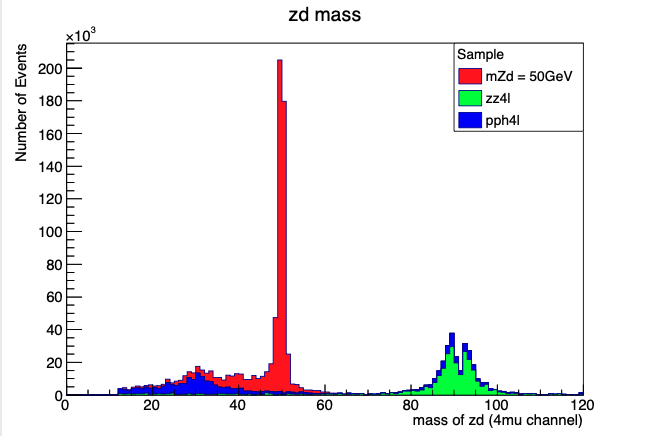# Normalize sample to BR, Luminosity & XS

hi
i am trying to normalize my samples to the standr model higgs boson production cross section 48.58pb and BR of 1.25e-4 and to the Luminosity of 30fb-1

1.here, i can only find the equation for normalization to be, xs * BR/nevents, and L*XS/nevents, sorry for my lame question but how do i include all three in 1 equation. XS BR and Luminosity

``````// normalize using scale to SM Higgs crossection & BR (total events 25k)
h_sig->Scale(45.58*(1.25e-4/25000));
h_bkg_zz4lep->Scale(45.58*(1.25e-4/25000));
h_bkg_pph4l->Scale(45.58*(1.25e-4/25000));

//number of signal and background events determined by integrating over region of interest
int nsig = h_sig->Integral(15,120);
cout << "signal nevent is = " << nsig << ".\n";

int nbkg_zz4lep = h_bkg_zz4lep->Integral(15, 120);
cout << "zz4lep nevent is = " << nbkg_zz4lep << ".\n";

int nbkg_pph4l = h_bkg_pph4l->Integral(15,120);
cout << "pph4lep nevent is = " << h_bkg_pph4l << ".\n";

double S = nsig;
double B = nbkg_zz4lep + nbkg_pph4l;

// calculate significance
double significance1 = 2*((sqrt(S+B))-(sqrt(B)));
cout << "significance1 = " << significance1 << ".\n";
double significance2 = sqrt((2*(S+B)*log(1.0+S/B)-2*S));
cout << "significance2 = " << significance2 << ".\n";
``````

this is my code so far, where i only scale to xs *BR/nevents, i ran this code and got this result

``````signal nevent is = 0.
zz4lep nevent is = 0.
pph4lep nevent is = 0x7fc2a6d8a620.
significance1 = 0.
significance2 = nan.
``````

clearly i am declaring something wrong,uploaded here is a stack of my histograms,clearly i am doing something wrong can you help me,

thanks

_ROOT Version:_6.22
_Platform:_mac catalina 10.15.16
_Compiler:Apple clang version 11.0.3 (clang-1103.0.32.29)
___hi if i scale it to it to xs and luminosity

`````` h_sig->Scale(48.58*250/25000);
h_bkg_zz4lep->Scale(48.58*250/25000);
h_bkg_pph4l->Scale(48.58*250/25000);
``````

i get

``````signal nevent is = 3135.
zz4lep nevent is = 1407.
pph4lep nevent is = 0x7fd7839d4420.
significance1 = 50.0069.
significance2 = 53.596.
``````

so i dont think the sytax

``````int nsig = h_sig->Integral(15,120);
``````

is wrong, i think the way im implementing BR of higgs 1.25e-4 here is wrong

``````// normalize using scale to SM Higgs crossection & BR (total events 25k)
h_sig->Scale(45.58*(1.25e-4/25000));
``````

does the BR need to be scale or some sort ? because it its too small, and of course this will lead to 0 as ourput

``````->Scale(XS*L*(BR/nevents));
``````->Scale(48.58*250*(1.25e-4/25000));